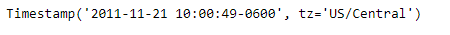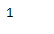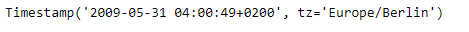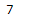# Python | Pandas Timestamp.isoweekday

• Last Updated : 14 Jan, 2019

Python is a great language for doing data analysis, primarily because of the fantastic ecosystem of data-centric python packages. Pandas is one of those packages and makes importing and analyzing data much easier.

Pandas` Timestamp.isoweekday()` function return the day of the week represented by the date. where days of the week are numbered from Monday being 1 to Sunday being 7.

Attention geek! Strengthen your foundations with the Python Programming Foundation Course and learn the basics.

To begin with, your interview preparations Enhance your Data Structures concepts with the Python DS Course. And to begin with your Machine Learning Journey, join the Machine Learning - Basic Level Course

Syntax :Timestamp.isoweekday()

Parameters : None

Return : weekday

Example #1: Use `Timestamp.isoweekday()` function to find the day of the week for the date in the given Timestamp object.

 `# importing pandas as pd``import` `pandas as pd`` ` `# Create the Timestamp object``ts ``=` `pd.Timestamp(year ``=` `2011``,  month ``=` `11``, day ``=` `21``,``                  ``hour ``=` `10``, second ``=` `49``, tz ``=` `'US/Central'``)`` ` `# Print the Timestamp object``print``(ts)`

Output :Now we will use the `Timestamp.isoweekday()` function to find the day of the week for the date in ts object.

 `# return the day of the week``ts.isoweekday()`

Output :As we can see in the output, the `Timestamp.isoweekday()` function has returned 1 indicating that the date in the given Timestamp object is Monday.

Example #2: Use `Timestamp.isoweekday()` function to find the day of the week for the date in the given Timestamp object.

 `# importing pandas as pd``import` `pandas as pd`` ` `# Create the Timestamp object``ts ``=` `pd.Timestamp(year ``=` `2009``, month ``=` `5``, day ``=` `31``,``                  ``hour ``=` `4``, second ``=` `49``, tz ``=` `'Europe/Berlin'``)`` ` `# Print the Timestamp object``print``(ts)`

Output :Now we will use the `Timestamp.isoweekday()` function to find the day of the week for the date in ts object.

 `# return the day of the week``ts.isoweekday()`

Output :As we can see in the output, the `Timestamp.isoweekday()` function has returned 7 indicating that the date in the given Timestamp object is Sunday.

My Personal Notes arrow_drop_up